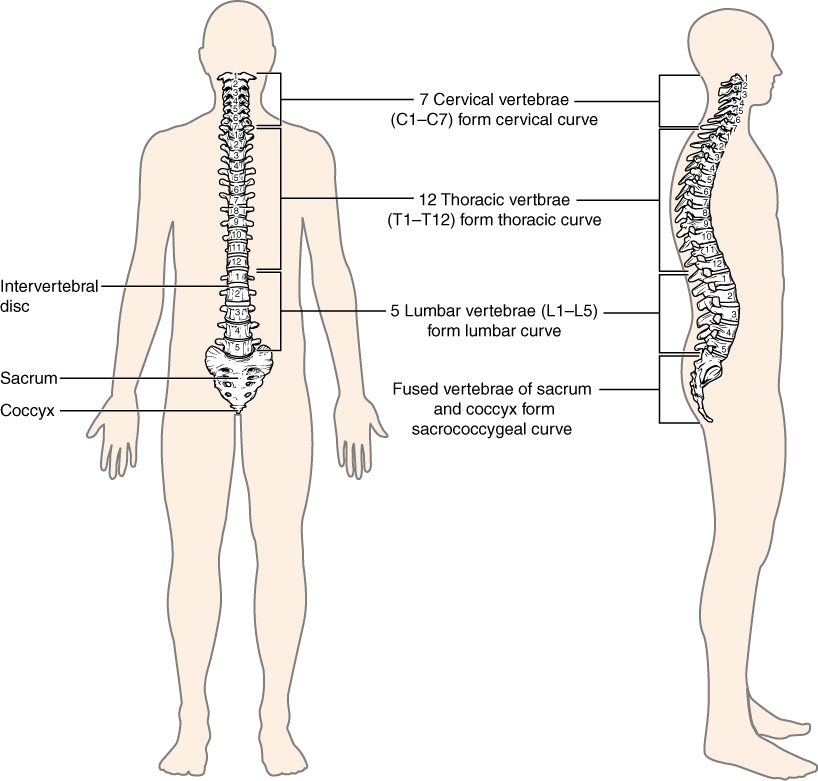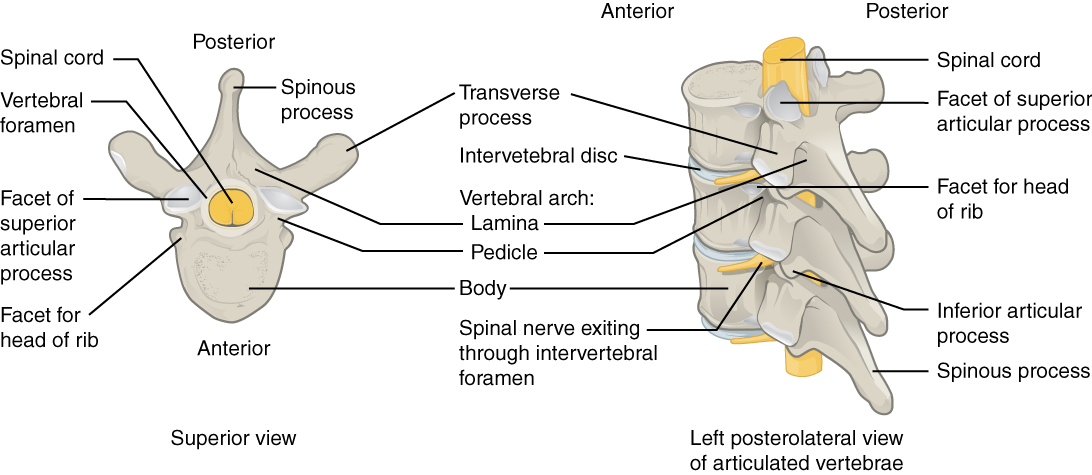# 0.8 Skeletal system module 9: the vertebral column

 Page 1 / 48
By the end of this section, you will be able to:
• Describe each region of the vertebral column and the number of bones in each region
• Discuss the curves of the vertebral column and how these change after birth
• Describe a typical vertebra and determine the distinguishing characteristics for vertebrae in each vertebral region and features of the sacrum and the coccyx
• Define the structure of an intervertebral disc
• Determine the location of the ligaments that provide support for the vertebral column

The vertebral column is also known as the spinal column or spine ( [link] ). It consists of a sequence of vertebrae (singular = vertebra) , each of which is separated and united by an intervertebral disc    . Together, the vertebrae and intervertebral discs form the vertebral column. It is a flexible column that supports the head, neck, and body and allows for their movements. It also protects the spinal cord, which passes down the back through openings in the vertebrae.The adult vertebral column consists of 24 vertebrae, plus the sacrum and coccyx. The vertebrae are divided into three regions: cervical C1–C7 vertebrae, thoracic T1–T12 vertebrae, and lumbar L1–L5 vertebrae. The vertebral column is curved, with two primary curvatures (thoracic and sacrococcygeal curves) and two secondary curvatures (cervical and lumbar curves).

## Regions of the vertebral column

The vertebral column originally develops as a series of 33 vertebrae, but this number is eventually reduced to 24 vertebrae, plus the sacrum and coccyx. The vertebral column is subdivided into five regions, with the vertebrae in each area named for that region and numbered in descending order. In the neck, there are 7 cervical vertebrae . The first vertebra articulates (forms a joint) with the skull. It is called the atlas    . The second vertebra is called the axis    . Below these are the 12 thoracic vertebrae , each of these vertebrae form a joint with a pair of ribs. The lower back contains the 5 lumbar vertebrae . The single sacrum    , which is also part of the pelvis, is formed by the fusion of five sacral vertebrae. Similarly, the coccyx    , or tailbone, results from the fusion of four small coccygeal vertebrae. However, the sacral and coccygeal fusions do not start until age 20 and are not completed until middle age.

## Curvatures of the vertebral column

Disorders associated with the curvature of the spine include kyphosis    (an excessive posterior curvature of the thoracic region), lordosis    (an excessive anterior curvature of the lumbar region), and scoliosis    (an abnormal, lateral curvature, accompanied by twisting of the vertebral column).

## General structure of a vertebra

Within the different regions of the vertebral column, vertebrae vary in size and shape, but they all follow a similar structural pattern. A typical vertebra will consist of a body , a vertebral foramen    , along with other processes. The body is the anterior portion of each vertebra and is the part that supports the body weight. Because of this, the vertebral bodies progressively increase in size and thickness going down the vertebral column. The bodies of adjacent vertebrae are separated and strongly united by an intervertebral disc    . ( [link] ). The large opening between the vertebral arch and body is the vertebral foramen, which contains the spinal cord. The vertebral foramina of all of the vertebrae align to form the vertebral (spinal) canal    , which serves as the bony protection and passageway for the spinal cord down the back.A typical vertebra consists of a body and a vertebral arch. The arch is formed by the paired pedicles and paired laminae. Arising from the vertebral arch are the transverse, spinous, superior articular, and inferior articular processes. The vertebral foramen provides for passage of the spinal cord. Each spinal nerve exits through an intervertebral foramen, located between adjacent vertebrae. Intervertebral discs unite the bodies of adjacent vertebrae.

## Regional modifications of vertebrae

In addition to the general characteristics of a typical vertebra described above, vertebrae also display characteristic size and structural features that vary between the different vertebral column regions. Thus, cervical vertebrae are smaller than lumbar vertebrae due to differences in the proportion of body weight that each supports. Thoracic vertebrae have sites for rib attachment, and the vertebrae that give rise to the sacrum and coccyx have fused together into single bones.

## Chapter review

The vertebral column forms the neck and back. The vertebral column originally develops as 33 vertebrae, but is eventually reduced to 24 vertebrae, plus the sacrum and coccyx. The vertebrae are divided into the cervical region (C1–C7 vertebrae), the thoracic region (T1–T12 vertebrae), and the lumbar region (L1–L5 vertebrae). The sacrum arises from the fusion of five sacral vertebrae and the coccyx from the fusion of four small coccygeal vertebrae. The vertebral column has four curvatures, the cervical, thoracic, lumbar, and sacrococcygeal curves. The thoracic and sacrococcygeal curves are primary curves retained from the original fetal curvature. The cervical and lumbar curves develop after birth and thus are secondary curves. The cervical curve develops as the infant begins to hold up the head, and the lumbar curve appears with standing and walking.

show that the set of all natural number form semi group under the composition of addition
explain and give four Example hyperbolic function
_3_2_1
felecia
⅗ ⅔½
felecia
_½+⅔-¾
felecia
The denominator of a certain fraction is 9 more than the numerator. If 6 is added to both terms of the fraction, the value of the fraction becomes 2/3. Find the original fraction. 2. The sum of the least and greatest of 3 consecutive integers is 60. What are the valu
1. x + 6 2 -------------- = _ x + 9 + 6 3 x + 6 3 ----------- x -- (cross multiply) x + 15 2 3(x + 6) = 2(x + 15) 3x + 18 = 2x + 30 (-2x from both) x + 18 = 30 (-18 from both) x = 12 Test: 12 + 6 18 2 -------------- = --- = --- 12 + 9 + 6 27 3
Pawel
2. (x) + (x + 2) = 60 2x + 2 = 60 2x = 58 x = 29 29, 30, & 31
Pawel
ok
Ifeanyi
on number 2 question How did you got 2x +2
Ifeanyi
combine like terms. x + x + 2 is same as 2x + 2
Pawel
x*x=2
felecia
2+2x=
felecia
Mark and Don are planning to sell each of their marble collections at a garage sale. If Don has 1 more than 3 times the number of marbles Mark has, how many does each boy have to sell if the total number of marbles is 113?
Mark = x,. Don = 3x + 1 x + 3x + 1 = 113 4x = 112, x = 28 Mark = 28, Don = 85, 28 + 85 = 113
Pawel
how do I set up the problem?
what is a solution set?
Harshika
find the subring of gaussian integers?
Rofiqul
hello, I am happy to help!
Abdullahi
hi mam
Mark
find the value of 2x=32
divide by 2 on each side of the equal sign to solve for x
corri
X=16
Michael
Want to review on complex number 1.What are complex number 2.How to solve complex number problems.
Beyan
yes i wantt to review
Mark
use the y -intercept and slope to sketch the graph of the equation y=6x
how do we prove the quadratic formular
Darius
hello, if you have a question about Algebra 2. I may be able to help. I am an Algebra 2 Teacher
thank you help me with how to prove the quadratic equation
Seidu
may God blessed u for that. Please I want u to help me in sets.
Opoku
what is math number
4
Trista
x-2y+3z=-3 2x-y+z=7 -x+3y-z=6
can you teacch how to solve that🙏
Mark
Solve for the first variable in one of the equations, then substitute the result into the other equation. Point For: (6111,4111,−411)(6111,4111,-411) Equation Form: x=6111,y=4111,z=−411x=6111,y=4111,z=-411
Brenna
(61/11,41/11,−4/11)
Brenna
x=61/11 y=41/11 z=−4/11 x=61/11 y=41/11 z=-4/11
Brenna
Need help solving this problem (2/7)^-2
x+2y-z=7
Sidiki
what is the coefficient of -4×
-1
Shedrak
how did you get the value of 2000N.What calculations are needed to arrive at it
Privacy Information Security Software Version 1.1a
Good
Got questions? Join the online conversation and get instant answers!

#### Get Jobilize Job Search Mobile App in your pocket Now!By Madison ChristianBy Lakeima RobertsBy Dakota BocanBy Stephen VoronBy OpenStaxBy OpenStaxBy Hannah ShethBy Cath YuBy Jessica CollettBy Jazzycazz Jackson